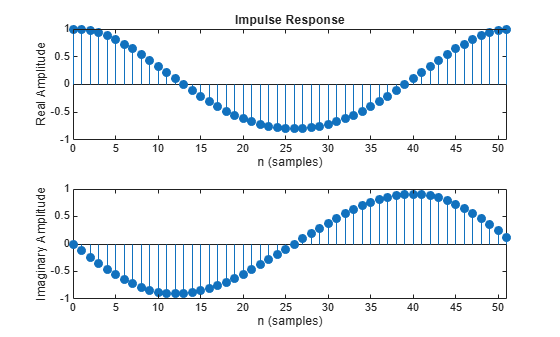# fftcoeffs

Frequency-domain coefficients

## Syntax

```c = fftcoeffs(hd) ```

## Description

`c = fftcoeffs(hd)` return the frequency-domain coefficients used when filtering with the `dfilt.fftfir` object. c contains the coefficients

## Examples

collapse all

This example demonstrates returning the FFT coefficients from the discrete-time filter hd.

```b = [0.05 0.9 0.05]; len = 50; hd = dfilt.fftfir(b,len); c=fftcoeffs(hd);```

Plot the impulse response of the coefficients.

`impz(c);`Watch now